# Rational Number In Decimal Representation

## Number system of Class 9

### TERMINATING DECIMAL:

In this a finite number of digit occurs after decimal i.e. 1/2= 0.5, 0.6875, 0.15 etc.

### NON-TERMINATING AND REPEATING (RECURRING DECIMAL):

In this a set of digits or a digit is repeated continuously.

e.g.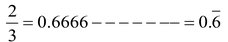.

e.g.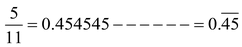.

### PROPERTIES OF RATIONAL NUMBER

If a,b,c are three rational numbers.

(i) Commutative property of addition. a + b = b + a

(ii) Associative property of addition (a+b)+c = a+(b+c)

(iii) Additive inverse a + (-a) = 0

0 is identity element, -a is called additive inverse of a.

(iv) Commutative property of multiplications a.b. = b.a.

(v) Associative property of multiplication (a.b).c = a.(b.c)

(vi) Multiplicative inverse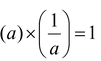1 is called multiplicative identity and 1/a is called multiplicative inverse of a or reciprocal of a.

(vii) Distributive property a.(b+c) = a.b + a.c

question Prove that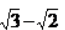is an irrational number.

Solution: Let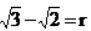where r be a rational number

Squaring both sides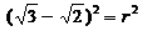⇒ 3 + 2 – 2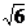=r2

⇒ 5 – 2=r2

Here, 5 –2is an irrational number but r2is a rational number.

∴ L.H.S. ≠ R.H.S.

Hence it contradicts our assumption thatis a rational number.

(b) Irrational Number in Decimal Form :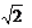= 1.414213 ...... i.e. it is not-recurring as well as non-terminating.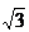= 1.732050807 ...... i.e. it is non-recurring as well as non-terminating.

question Insert an irrational number between 2 and 3.

Solution: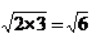question Find two irrational number between 2 and 2.5.

Solution: 1st Method :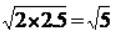Since there is no rational number whose square is 5. So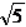is irrational..

Also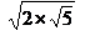is a irrational number.

2nd Method: 2.101001000100001.... is between 2 andand it is non-recurring as well as non-terminating.

Also, 2.201001000100001......... and so on.

question Find two irrational number betweenand.

Solution: 1st Method :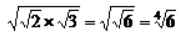Irrational number betweenand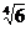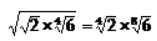2nd Method : As= 1.414213562 ...... and= 1.732050808......

As ,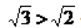andhas 4 in the 1st place of decimal whilehas 7 is the 1st place of decimal.

∴ 1.501001000100001......., 1.601001000100001...... etc. are in betweenandquestion Find two irrational number between 0.12 and 0.13.

Solution: 0.1201001000100001......., 0.12101001000100001 .......etc.

question Find two irrational number between 0.3030030003..... and 0.3010010001 .......

Solution: 0.302020020002...... 0.302030030003.... etc.

question Find two rational number between 0.2323323332..... and 0.252552555255552.......

Solution: 1st place is same 2.

2nd place is 3 & 5.

3rd place is 2 in both.

4th place is 3 & 5.

Let a number = 0.25, it falls between the two irrational number.

Also a number = 0.2525 an so on.